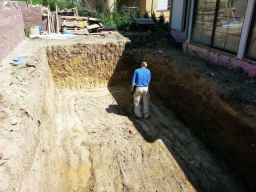# One-quarter 58883

The workers dug one-quarter of the excavation on the first day, half the rest on the second day, and the remaining 15 m on the third day. How many meters did they dig on the first and second day?

a =  10 m
b =  15 m

### Step-by-step explanation:Did you find an error or inaccuracy? Feel free to write us. Thank you!

Tips for related online calculators
Need help calculating sum, simplifying, or multiplying fractions? Try our fraction calculator.
Do you have a linear equation or system of equations and looking for its solution? Or do you have a quadratic equation?
Do you want to convert length units?